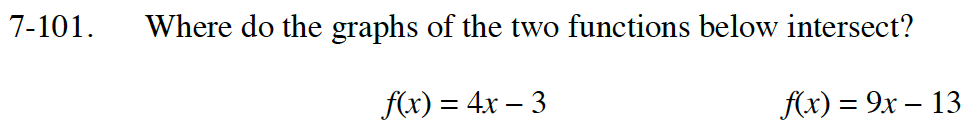Home > CC4 > Chapter 7 > Lesson 7.2.2 > Problem7-101

7-101.
1. Where do the graphs of the two functions below intersect? Homework Help ✎

f(x) = 4x – 3 f(x) = 9x – 13There are many ways to solve this problem. You could use a table, a graph, the Equal Values method, or the Substitution method.